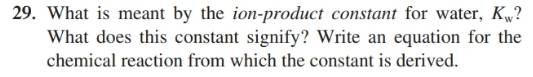Chapter 16, Problem 29QAP### Introductory Chemistry: A Foundati...

9th Edition
Steven S. Zumdahl + 1 other
ISBN: 9781337399425

#### Solutions

Chapter
Section### Introductory Chemistry: A Foundati...

9th Edition
Steven S. Zumdahl + 1 other
ISBN: 9781337399425
Textbook Problem
5 views

# . What is meant by the iou-product constant for water, KW? What does this constant signify? Write an equation for the chemical reaction from which the constant is derived.Interpretation Introduction

Interpretation:

The ion-product constant for water Kw should be defined along with the significance of this constant. An equation for a chemical reaction should be determined from which Kw is derived.

Concept Introduction:

Water is an amphoteric in nature implies it can act as an acid as well as base.

Ionization of water is given as:

H2O(l)+H2O(l)H+(aq)+OH(aq)

Here, one water molecule is act as an acid by accepting proton and another molecule of water act as a base by donating proton.

At 25°C, the actual concentrations of the products are:

[H+][OH]=1×1014

The mathematical product of both [H+] and [OH] concentrations are always constant known as ion product constant ( Kw )

[H+][OH]=1×1014=Kw.

Explanation

H2O(l)+H2O(l)H+(aq)+OH(aq)

Ion-product product constant for water is equal to the product of hydrogen ion concentration and hydroxide ion concentration and the value of ion- product constant is equal to 1×1014 at 25°C. This is denoted by Kw

[H+][OH]=1×1014=Kw

With increase in temperature, concentration of hydrogen ion and concentration of hydroxide increases and due to increase in concentration of both ions, value of ion- product constant also increases

### Still sussing out bartleby?

Check out a sample textbook solution.

See a sample solution

#### The Solution to Your Study Problems

Bartleby provides explanations to thousands of textbook problems written by our experts, many with advanced degrees!

Get Started

#### Find more solutions based on key concepts